# 深度学习: Regularization (正则化)

## 正则化手段

L1正则化、L2正则化、Elastic网络正则化、最大范数约束、随机失活、使用验证集。

## L1正则化

${l}_{1}=\lambda ||\omega |{|}_{1}=\sum _{i}|{\omega }_{i}|$

$\lambda$$\lambda$ ：取值越大，则对模型复杂度的 约束程度 越大。

## L2正则化

L2正则化 的 别名 权重衰减 (weight decay) 岭回归 (ridge regression)

${l}_{2}=\frac{1}{2}\lambda ||\omega |{|}_{2}^{2}$

Note

• 现在正则化一般用L2正则化，因为其便于求导，从而方便优化；
• L1正则化求导不易，大家一般不用。

## Elastic网络正则化

Elastic网络正则化 = L1正则化 + L2正则化

L1正则化 约束参数量级 + 稀疏化参数（可以造就更多的0值）
L2正则化 （只能）约束参数量级

${l}_{Elastic}={l}_{1}+{l}_{2}={\lambda }_{1}||\omega |{|}_{1}+{\lambda }_{2}||\omega |{|}_{2}^{2}$

## 最大范数约束

$||\omega |{|}_{2}

$c$$c$：一般取 ${10}^{3}\sim {10}^{4}$$10^3 \sim 10^4$ 数量级。

## 随机失活

Note :
- 只针对 全连接层 进行操作；
- 训练阶段和测试阶段的操作不同。

### 训练阶段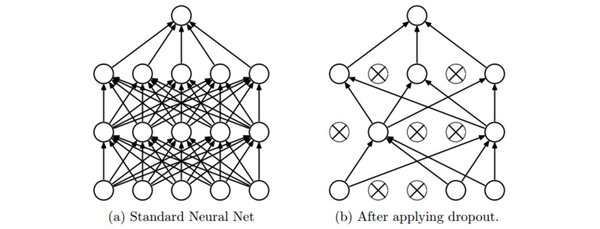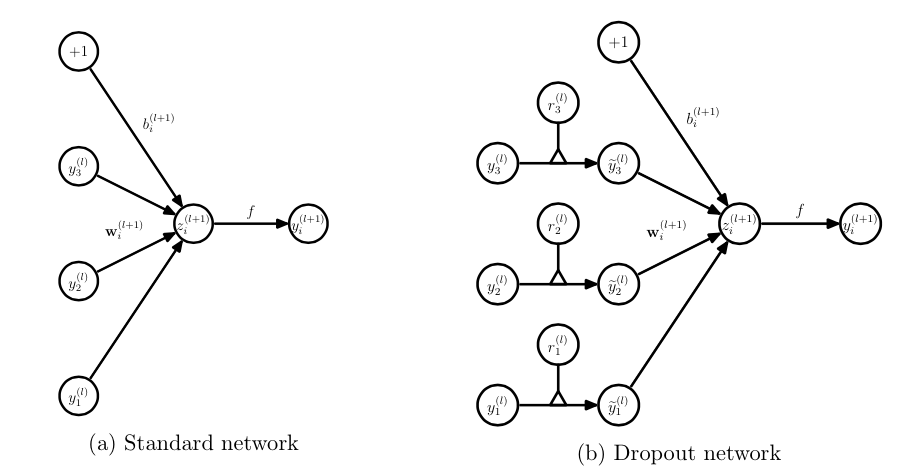• 没有dropout的神经网络：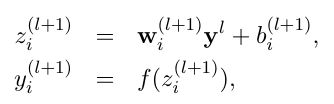• 有dropout的神经网络：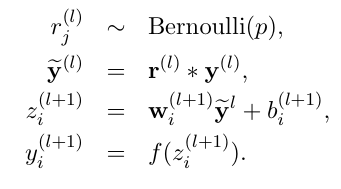Tensorflow 中的 dropout API 如下：

tf.nn.dropout

# coding=utf-8

import tensorflow as tf
import numpy as np

inputs = np.random.uniform(-10, 10, size=[3, 3])
features = tf.placeholder_with_default(input=inputs, shape=[3, 3])

# 随机失活
output_dropout = tf.nn.dropout(features, keep_prob=0.5)

with tf.Session() as sess:
print '\nfeatures :\n', sess.run(features)
print '\n----------\n'
print '\ndropout :\n', sess.run(output_dropout)
features :
[[ 0.53874537 -3.09047282 -2.88714205]
[-1.92602402 -1.56025457  3.64309646]
[-9.13147387  8.37367913 -7.9849204 ]]

----------

dropout :
[[  0.          -6.18094565  -5.77428411]
[ -0.          -3.12050914   7.28619293]
[-18.26294775  16.74735827  -0.        ]]

### 测试阶段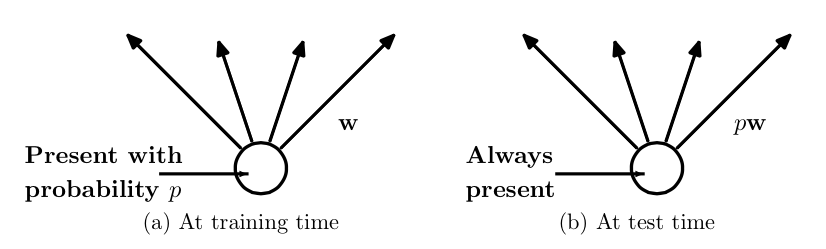## 使用验证集